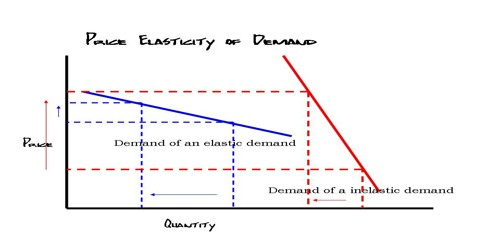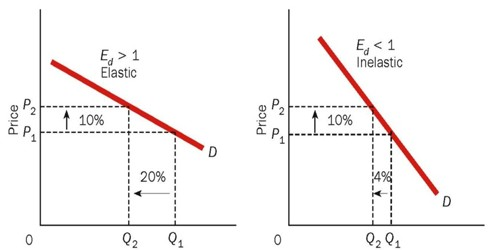Economics

# Concept of Price Elasticity of DemandConcept of Price Elasticity of Demand

The price elasticity of demand measures the degree of responsiveness of quantity demanded for a certain commodity to the change in its price. It measures the change in demand for a good in response to a change in price. Price elasticity of demand is a measure of the relationship between a change in the quantity demanded of a particular good and a change in its price. Price elasticity of demand is a term in economics often used when discussing price sensitivity. In other words, the price elasticity of demand is defined as the ‘ratio of percentage change in the quantity demanded to the percentage change in price. It can be expressed as follows:

Price elasticity of demand (ep) = Percentage change in quantity of demand / Percentage change in price

Where, ep = Coefficient of price elasticity of demand.

The price elasticity of demand is always negative due to the inverse relationship between the price and quantity demanded. But for the sake of simplicity in understanding the magnitude of response of quantity demanded to the change in the price we ignore the negative sign and take into account only the numerical value of the price elasticity of demand.We use the word “coefficient” to describe the values for price elasticity of demand

• If Ped = 0 demand is perfectly inelastic – demand does not change at all when the price changes – the demand curve will be vertical.
• If Ped is between 0 and 1 (i.e. the % change in demand from A to B is smaller than the percentage change in price), then demand is inelastic.
• If Ped = 1 (i.e. the % change in demand is exactly the same as the % change in price), then demand is unit elastic. A 15% rise in price would lead to a 15% contraction in demand leaving total spending the same at each price level.
• If Ped > 1, then demand responds more than proportionately to a change in price i.e. demand is elastic. For example if a 10% increase in the price of a good leads to a 30% drop in demand. The price elasticity of demand for this price change is –3.

Price elasticity of demand is calculated by dividing the proportionate change in quantity demanded by the proportionate change in price. Proportionate (or percentage) changes are used so that the elasticity is a unit-less value and does not depend on the types of measures used (e.g. kilograms, pounds, etc).

As an example, if a 2% increase in price resulted in a 1% decrease in quantity demanded, the price elasticity of demand would be equal to approximately 0.5. It is not exactly 0.5 because of the specific definition for elasticity uses the average of the initial and final values when calculating percentage change. When the elasticity is calculated over a certain arc or section of the demand curve, it is referred to as the am elasticity and is defined as the magnitude (absolute value) of the following:

Information Source: